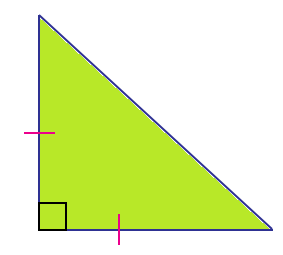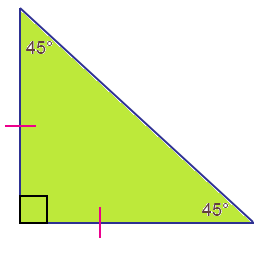# Isosceles Right Triangle

A right triangle is a triangle in which exactly one angle measures 90 degrees. Since the sum of the measures of angles in a triangle has to be 180 degrees, it is evident that the sum of the remaining two angles would be another 90 degrees. The two perpendicular sides are called the legs of a right triangle, and the longest side that lies opposite the 90-degree is called the hypotenuse of a right triangle. A right triangle can be scalene (having all three sides of different length) or isosceles (having exactly two sides of equal length). It can never be an equilateral triangle. In this article, you are going to study the definition, area, and perimeter of an isosceles right triangle in detail.

## Isosceles Right Triangle Definition

An Isosceles Right Triangle is a right triangle that consists of two equal length legs. Since the two legs of the right triangle are equal in length, the corresponding angles would also be congruent. Thus, in an isosceles right triangle, two legs and the two acute angles are congruent.Since it is a right triangle, the angle between the two legs would be 90 degrees, and the legs would obviously be perpendicular to each other.

## Isosceles Right Triangle Formula

The most important formula associated with any right triangle is the Pythagorean theorem. According to this theorem, the square of the hypotenuse is equal to the sum of the squares of the other two sides of the right triangle. Now, in an isosceles right triangle, the other two sides are congruent. Therefore, they are of the same length “l”. Thus, the hypotenuse measures h, then the Pythagorean theorem for isosceles right triangle would be:

(Hypotenuse)2 = (Side)2 + (Side)2

h2 = l2 + l2

h2 = 2l2

Also, two congruent angles in isosceles right triangle measure 45 degrees each, and the isosceles right triangle is:## Area of an Isosceles Right Triangle

As we know that the area of a triangle (A) is ½ bh square units

Where

b is the base of the triangle

h is the altitude of the triangle

In an isosceles right triangle, two legs are of equal length. Let us say that they both measure “l” then the area formula can be further modified to:

Area, A = ½ (l × l)

A = ½ l2

Area of an Isosceles Right Triangle = l2/2 square units.

Where

l is the length of the congruent sides of the isosceles right triangle

## Perimeter of an Isosceles Right Triangle

The perimeter of any plane figure is defined as the sum of the lengths of the sides of the figure. For a triangle, the perimeter would be the sum of all the sides of the triangle. Thus, the perimeter a triangle with side lengths a, b, and c, would be:

Perimeter of a triangle = a + b + c units

In an isosceles right triangle, we know that two sides are congruent. Suppose their lengths are equal to l, and the hypotenuse measures h units. Thus the perimeter of an isosceles right triangle would be:

Perimeter = h + l + l units

Therefore, the perimeter of an isosceles right triangle P is h + 2l units.

Where

h is the length of the hypotenuse side

l is the length of the adjacent and opposite sides

### Isosceles Right Triangle Example

Question:

Find the area and perimeter of an isosceles right triangle whose hypotenuse side is 10 cm.

Solution:

Given:

Length of the hypotenuse side, h = 10 cm

We know that, h2 = 2l2

Substitute the value of “h” in the above formula:

102 = 2l2

100= 2l2

l2 = 100/2

l2 = 50

Therefore, l = √50 = 5√2 cm

Therefore, the length of the congruent legs is 5√2 cm.

So, the area of an isosceles right triangle, A = l2/2

A = (5√2)2/2

A = (25 x 2)/2

A = 25

Therefore, the area of an isosceles right triangle = 25 cm2

The perimeter of an isosceles right triangle, p = h+ 2l units

P = 10 + 2( 5√2)

P = 10 + 10√2

Substituting √2 = 1.414,

P = 10 + 10(1.414)

P = 10 + 14.14

P = 24.14

Therefore, the perimeter of an isosceles right triangle is 24.14 cm.

Register with BYJU’S – The Learning App and also download the app to read all Maths-related topics and explore videos to learn with ease.

Test your knowledge on Isosceles Right Triangle